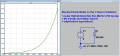# How do I calculate the voltage across a diode?

#### Swaysceptile

Joined Oct 28, 2021
16
How do I calculate the voltage across a diode using ideal, practical, and complete models? What are the steps? I can't find any good tutorials/resources on the internet.

I know the formula, but kinda confused with those many resistors.

Hope someone could help

#### Papabravo

Joined Feb 24, 2006
18,120
You don't calculate the voltage across the diode. You assume a typical value of between 0.6 and 0.7 Volts. Then you solve for the currents and see if they match your assumptions. When the diode conducts the actual voltage should be close to the assumed value because of the slope of the exponential curve.See how steep the curve is getting from 690 to 750 millivolts of forward bias.

#### Swaysceptile

Joined Oct 28, 2021
16
You don't calculate the voltage across the diode. You assume a typical value of between 0.6 and 0.7 Volts. Then you solve for the currents and see if they match your assumptions. When the diode conducts the actual voltage should be close to the assumed value because of the slope of the exponential curve.

View attachment 251410

See how steep the curve is getting from 690 to 750 millivolts of forward bias.
Right now we're only on the conceptual part, like a physics problem. But thank you I will try getting into what you said

#### MrChips

Joined Oct 2, 2009
25,930
This is a tricky question to solve analytically.
You have to make some assumptions, i.e. what is the diode forward current and forward voltage?

1) Firstly, remove the 4.7kΩ and diode from the circuit. Calculate the voltage available at the node that goes to the diode.
This will tell you if there is enough voltage to forward bias the diode.

2) Secondly, replace the diode with a short to ground (with the 4.7kΩ series resistor in place). Do the above calculation.

3) Repeat step (2) and determine the current through the 4.7kΩ series resistor. Use the graph in post #2 above and estimate the diode forward voltage.

4) Do the entire circuit analysis with this estimation of the diode forward voltage.

Or do the entire exercise and assume that the diode forward voltage is 0.63V.

•hobbyist

#### neonstrobe

Joined May 15, 2009
181
Are three different solutions needed : ideal, practical, and complete models
Ideal diode- conducts for any voltage (a-k) >0 and not at all for V <=0.
Easy: short the diode out. Calculate the currents/voltages in the resistors.
Practical diode - choose a typical forward voltage as others have said e.g. 0.65V and calculate the currents with that assumption.
Complete model - that's tricky. It needs an electrical model of the diode and will have to be solved using iterative methods.
Is that something you have been given - a "complete" model of a diode?
Have you heard of Newton (or Newton-Raphson) iteration?